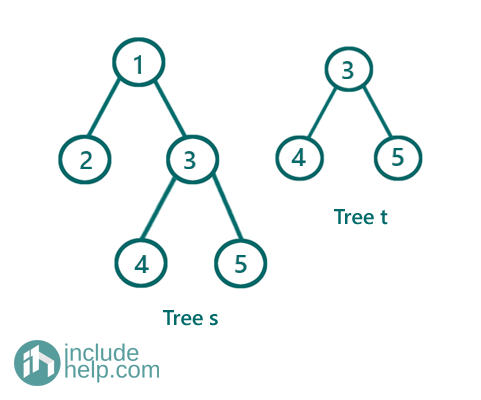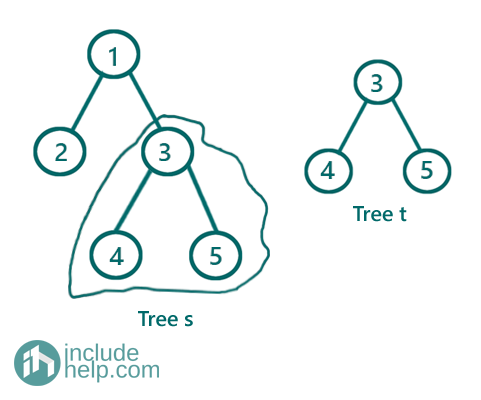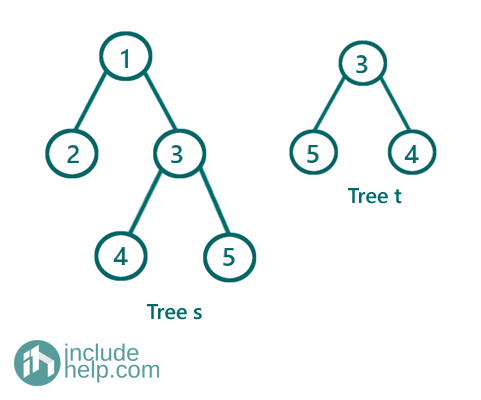# Check if a binary tree is a subtree of another binary tree

In this article, we are going to see how to find whether a binary tree is a subtree of another binary tree or not? Firstly, we have discussed the naïve approach and then we gradually moved to a better solution.
Submitted by Radib Kar, on August 12, 2020

Prerequisite: Check if two trees are identical or not

A binary tree t can be a subtree of another binary tree s if any subtree of tree s is identical with the tree t.

Example and Explanation:

1) When we find a subtree to be identical with the other treeIn the above example, below is the subtree marked which is identical with the tree t.It's clear that the marked subtree is identical with the tree t and that's why we can say tree t is a subtree of tree s.

2) When we don't find any subtree to be identical with the other treeIn the above example, we can't find any subtree identical to tree t.

Solution:

1) Naïve approach

Below is the naïve approach where we implement the above definition, i.e., we check for each subtree of s whether similar to tree t or not.

For that, we have used level order traversal to process with each subtree. At level order traversal, each current node is the root of the subtree to be checked. To follow how to check whether two trees are identical or not?

Below is the implementation of the naïve approach.

```#include <bits/stdc++.h>
using namespace std;

// tree node is defined
class TreeNode {
public:
int val;
TreeNode* left;
TreeNode* right;
TreeNode(int data)
{
val = data;
left = NULL;
right = NULL;
}
};

//checking whether two trees are same or not
bool isSubtreerecur(TreeNode* s, TreeNode* t)
{
if (!s && !t)
return true;
if (!s || !t)
return false;

if (s->val == t->val)
return isSubtreerecur(s->left, t->left) && isSubtreerecur(s->right, t->right);
else
return false;
}

bool isSubtree(TreeNode* s, TreeNode* t)
{
if (!s && !t)
return true;
if (!s || !t)
return false;

//do a level order traversal and from each node
//check if the tree rooted by current node is same
//with the given tree t or not
//if they are same then if's guranteed that the
//given tree t is a subtree of the first tree s
queue<TreeNode*> q;
q.push(s);

while (!q.empty()) {
TreeNode* temp = q.front();
q.pop();
//if current value is same as the root value
//of t then only will will check
if (temp->val == t->val && isSubtreerecur(temp->left, t->left) && isSubtreerecur(temp->right, t->right))
return true;
if (temp->left)
q.push(temp->left);
if (temp->right)
q.push(temp->right);
}
return false;
}

int main()
{
//building the tree like example 1
TreeNode* s = new TreeNode(1);

s->left = new TreeNode(2);
s->right = new TreeNode(3);
s->right->left = new TreeNode(4);
s->right->right = new TreeNode(5);

TreeNode* t = new TreeNode(3);
t->left = new TreeNode(4);
t->right = new TreeNode(5);

if (isSubtree(s, t))
cout << "In example 1, tree t is subtree of tree s\n";
else
cout << "In example 1, tree t is not similar to any subtree of tree s\n";

//building the tree like example 2
s = new TreeNode(1);
s->left = new TreeNode(2);
s->right = new TreeNode(3);
s->right->left = new TreeNode(4);
s->right->right = new TreeNode(5);

t = new TreeNode(3);
t->left = new TreeNode(5);
t->right = new TreeNode(4);

if (isSubtree(s, t))
cout << "In example 2, tree t is subtree of tree s\n";
else
cout << "In example 2, tree t is not similar to any subtree of tree s\n";

return 0;
}
```

Output:

```In example 1, tree t is subtree of tree s
In example 2, tree t is not similar to any subtree of tree s
```

The time complexity for the above implementation is O(n*m) where n be the size of tree s and m be the size of tree t, considering the worst-case as we are checking for each subtree equal or not with the tree t.

2) Efficient approach

This is an efficient approach based on serialization. We serialize both the tree into a string by preorder traversals. First, we serialize the second tree, tree t, and then while serializing tree s we check whether any subtree has the same serialization as of tree t or not, below is the detailed implementation of the above idea.

```#include <bits/stdc++.h>
using namespace std;

// tree node is defined
class TreeNode {
public:
int val;
TreeNode* left;
TreeNode* right;
TreeNode(int data)
{
val = data;
left = NULL;
right = NULL;
}
};

//serialization by preorder traversal for tree s
//we store each subtree in a set
//to check whether it's same as t or not
//we serialize the tree to store
//it's an one-pass algorithm
string preorder_for_s(TreeNode* root, bool& ans, string& t)
{
//if ans is true no need to process any more
if (ans)
return "?";
if (!root)
return "#";

//serialize the current subtree
string cur = to_string(root->val) + "," + preorder_for_s(root->left, ans, t) + "," + preorder_for_s(root->right, ans, t);

//check if it's the same as the serialization of tree t
if (cur == t) {
ans = true;
return "?";
}
return cur;
}

//serialization of tree t by preorder traversal
string preorder_for_t(TreeNode* root)
{
if (!root)
return "#";
string cur = to_string(root->val) + "," + preorder_for_t(root->left) + "," + preorder_for_t(root->right);
return cur;
}

bool isSubtree(TreeNode* s, TreeNode* t)
{
string q = preorder_for_t(t);
bool ans = false;
preorder_for_s(s, ans, q);
return ans;
}

int main()
{
//building the tree like example 1
TreeNode* s = new TreeNode(1);
s->left = new TreeNode(2);
s->right = new TreeNode(3);
s->right->left = new TreeNode(4);
s->right->right = new TreeNode(5);

TreeNode* t = new TreeNode(3);
t->left = new TreeNode(4);
t->right = new TreeNode(5);

if (isSubtree(s, t))
cout << "In example 1, tree t is subtree of tree s\n";
else
cout << "In example 1, tree t is not similar to any subtree of tree s\n";

//building the tree like example 2
s = new TreeNode(1);
s->left = new TreeNode(2);
s->right = new TreeNode(3);
s->right->left = new TreeNode(4);
s->right->right = new TreeNode(5);

t = new TreeNode(3);
t->left = new TreeNode(5);
t->right = new TreeNode(4);

if (isSubtree(s, t))
cout << "In example 2, tree t is subtree of tree s\n";
else
cout << "In example 2, tree t is not similar to any subtree of tree s\n";

return 0;
}
```

Output:

```In example 1, tree t is subtree of tree s
In example 2, tree t is not similar to any subtree of tree s
```

In the above implementation, we did in only one pass and the time complexity is O (n+m).

What's New (MCQs)

Top Interview Coding Problems/Challenges!

Languages: » C » C++ » C++ STL » Java » Data Structure » C#.Net » Android » Kotlin » SQL
Web Technologies: » PHP » Python » JavaScript » CSS » Ajax » Node.js » Web programming/HTML
Solved programs: » C » C++ » DS » Java » C#
Aptitude que. & ans.: » C » C++ » Java » DBMS
Interview que. & ans.: » C » Embedded C » Java » SEO » HR
CS Subjects: » CS Basics » O.S. » Networks » DBMS » Embedded Systems » Cloud Computing
» Machine learning » CS Organizations » Linux » DOS
More: » Articles » Puzzles » News/Updates

© https://www.includehelp.com some rights reserved.